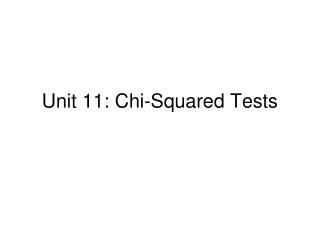DownloadDownload PresentationUnit 11: Chi-Squared Tests

# Unit 11: Chi-Squared Tests

Download Presentation## Unit 11: Chi-Squared Tests

- - - - - - - - - - - - - - - - - - - - - - - - - - - E N D - - - - - - - - - - - - - - - - - - - - - - - - - - -
##### Presentation Transcript

1. Unit 11: Chi-Squared Tests

2. Type I and type II errors • A “type I” error in a sig test is to reject H0 when it is true. • Many “discoveries” are type I errors • By def, α-value is P(type I error | H0 is true) • A “type II” error is to fail to reject H0 when it is false. • We never “accept H0” – no science is exactly right. (E.g., Einstein corrected Newton.)

3. Example: α-level and type I or II • Flip a coin, get 8 heads. With an H0 of a fair coin, P(count ≥ 8) = [C(10,8) + C(10,9) + C(10,10)] / 210≈ 5.5% • So with α = 10%, we reject null, while • with α = 5%, we do not reject null. • Therefore, if the coin is fair, 10% makes a type I error, and 5% yields the correct answer, • while if the coin is unfair, 10% yields the correct answer, and 5% makes a type II error.

4. The “power” of a test is P(no type II error | H0 is false) = 1 - P(type II | H0 false), but ... • ... we can’t compute the power because it depends on how false H0 is, and usually we don’t even know whether it is false. • Ex: Test a coin for fairness (H0 : P(head) = 0.5) with 20 flips. With α = 5%, test says coin unfair if #heads <= 5 or >= 15.Sample (binomial) distributions whenP(head) = 0.6 and 0.7: P(type II error) = 87% and 58% respectively.

5. Another example: An oracle speaks • Valesky vs. Brown for State Senate, interviewing 400 people. • H0: p = 50% of voters for Val; Ha: p > 50% • EV of (sample) % = .5, SE of % = √[.5(.5)/400] = .025 • For sig, need z = ((#/400)-.5)/.025 ≥ 1.96, i.e., # ≥ 220 • Oracle reveals true p = 52%: EV = .52, SE = √(.52(.48)/400) = .025 : P(Type II error) = P(z ≤ ((220/400)-.52)/.025 = 1.2) = 89% • Oracle reveals true p = 55%: EV = .55, SE = √(.55(.45)/400) = .025 : P(Type II error) = P(z ≤ ((220/400)-.55)/.025 = 0) = 50%

6. Still, … • As P(Type I error) [rejecting H0 when you shouldn’t] goes up -- maybe by picking a larger α or using a larger sample -- P(Type II error) [failing to reject H0 when you should] goes down, and v.v.